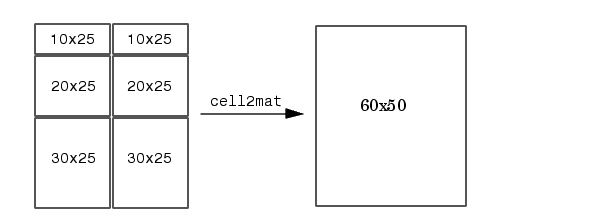MATLAB Function Referencecell2mat

Convert cell array of matrices to single matrix

Syntax

• ```m = cell2mat(c)
```

Description

```m = cell2mat(c) ``` converts a multidimensional cell array `c` with contents of the same data type into a single matrix, `m`. The contents of `c` must be able to concatenate into a hyperrectangle. Moreover, for each pair of neighboring cells, the dimensions of the cells' contents must match, excluding the dimension in which the cells are neighbors.

The example shown below combines matrices in a 3-by-2 cell array into a single 60-by-50 matrix:

• ```cell2mat(c)```

Remarks

The dimensionality (or number of dimensions) of `m` will match the highest dimensionality contained in the cell array.

`cell2mat` is not supported for cell arrays containing cell arrays or objects.

Examples

Combine the matrices in four cells of cell array `C` into the single matrix, `M`:

• ```C = { [2 3 4]; [5; 9] [6 7 8; 10 11 12]}
C =
[         1]    [1x3 double]
[2x1 double]    [2x3 double]

C{1,1}                     C{1,2}
ans =                      ans =
1                          2     3     4

C{2,1}                     C{2,2}
ans =                      ans =
5                          6     7     8
9                         10    11    12

M = cell2mat(C)
M =
1     2     3     4
5     6     7     8
9    10    11    12
```

See Also

`mat2cell`, `num2cell`

© 1994-2005 The MathWorks, Inc.